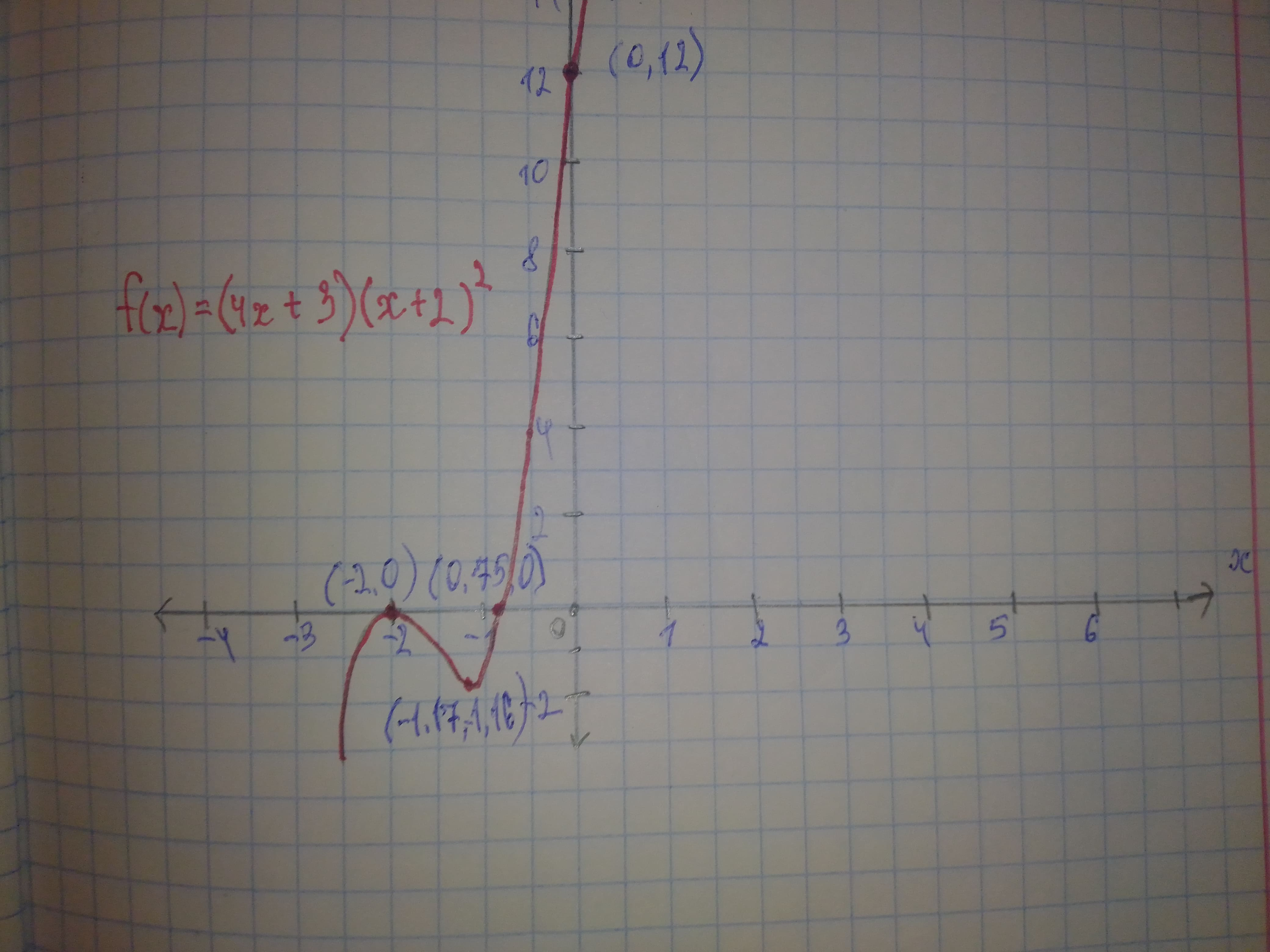# Graph each polynomial function. Factor first if the expression is not in factored form. f(x)=(4x+3)(x+2)^{2}Nannie Mack 2021-01-10 Answered
Graph each polynomial function. Factor first if the expression is not in factored form. $f\left(x\right)=\left(4x+3\right){\left(x+2\right)}^{2}$
You can still ask an expert for help

• Questions are typically answered in as fast as 30 minutes

Solve your problem for the price of one coffee

• Math expert for every subject
• Pay only if we can solve itAlannej

Step 1 $f\left(x\right)=\left(4x+3\right){\left(x+2\right)}^{2}$ The function has two zeros $-\frac{3}{4},-2$ So, the graph of f(x) crosses the x-axis at $\left(\frac{4}{3},0\right)$ and $\left(-2,0\right)$ To find the y-intercept, substitute 0 for x in f(x) $f\left(x\right)=\left(4x+3\right){\left(x+2\right)}^{2}$
$f\left(0\right)=\left(4\left(0\right)+3\right){\left(0+2\right)}^{2}$ Substitute 0 for x Simplify $=\left(3\right){\left(2\right)}^{2}$
$=12$ So, the function f(x) crosses the y-axis at (0,12) Step 2 $4x\cdot {\left(x\right)}^{2}=4{x}^{3}$ The leading coefficient is 4 Since the leading coefficient is positive and the function f(x) of degree 3 (odd degree) So, the end behavior is $x\to \mathrm{\infty },f\left(x\right)\to \mathrm{\infty }$
$x\to -\mathrm{\infty },f\left(x\right)\to -\mathrm{\infty }$ See the graph below